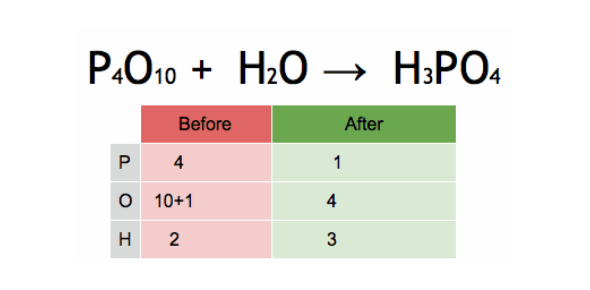# Balancing Equations And Chemical Reactions: Quiz

10 Questions | Total Attempts: 408SettingsWhen it comes to chemistry, students are expected to understand how to balance equations and chemical reactions. When mixed, different elements form a solution, and the changes can either be physical or chemical. Test your understanding of chemical reactions and solutions by taking the quiz below and check your notes for solutions after.

• 1.
A reaction that causes a chemical change is called _____.
• A.

Physical change

• B.

Chemical reaction

• C.

A flare

• D.

Light

• 2.
Reactants and products are both parts of _____.
• A.

Chemical equations

• B.

Math

• C.

Nuclear fission

• D.

Conservation of mass

• 3.
The two types of numbers in chemical equations are called _____.
• A.

Exponents and coefficients

• B.

• C.

Superscripts and subscripts

• D.

Coefficients and subscripts

• 4.
Coefficients tell us how many _____are in the equation.
• A.

Chemicals

• B.

Molecules

• C.

Atoms

• D.

Reactions

• 5.
Subscripts tell us how many _____ are in the equation.
• A.

Chemicals

• B.

Molecules

• C.

Atoms

• D.

Reactions

• 6.
Endothermic reactions ____.
• A.

Absorb heat energy

• B.

Release heat energy

• C.

Take place only in humans

• D.

Cannot be written

• 7.
Exothermic reactions _____.
• A.

Absorb heat energy

• B.

Release heat energy

• C.

Take place only in humans

• D.

Cannot be written

• 8.
If energy is written on the reactants side of an equation, it likely means that ____.
• A.

We need to mix energy with the other elements

• B.

The law of conservation of mass

• C.

Activation energy is required to start the reaction

• D.

• 9.
The energy released in reactions can be released in all of the following ways except _____.
• A.

Sound

• B.

In food

• C.

Heat

• D.

Light

• 10.
All of the following can affect the rate of a chemical reaction except_____.
• A.

Color

• B.

Concentration

• C.

Temperature

• D.

Surgace area

Related TopicsBack to top